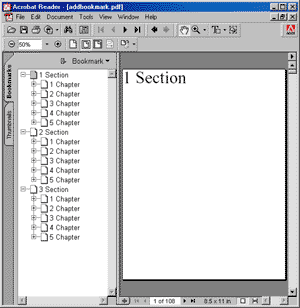AddBookmark FunctionAdds a bookmark pointing to the current page.Syntax

 ```int AddBookmark(string path, bool open); ``` ```Function AddBookmark(path As String, open As Boolean) As Integer ```Params

 Name Description path The path to the bookmark. open Whether the bookmark should be displayed open (expanded) or closed (collapsed). return The Object ID of the newly added Bookmark Object.Notes

Adds a bookmark which points to the current page.

Bookmarks are specified as paths in much the same way as file paths. Headings and subheadings are separated by a backslash character. For example:

"1. Introduction\1.1 Aim and Methods\1.1.3 Diagrams"

To add multiple bookmarks all referring to the same page simply call the AddBookmark function multiple times.

This function returns the Object ID of the newly added Bookmark Object.

 Hyperlinks. Sometimes you may wish to insert bookmarks which link to an external web page specified using a URI or URL. You can do this using hyperlink code of the following form.   ```static int AddBookmarkToUri(Doc doc, string bookmark, string uri) {   int id = doc.AddBookmark(bookmark, true);   doc.SetInfo(id, "/Dest:Del", ""); // remove link to page and add link to url   doc.SetInfo(id, "/A", "<< /Type /Action /S /URI /URI () >>");   doc.SetInfo(id, "/A/URI:Text", uri);   return id; } ``` ```Private Shared Function AddBookmarkToUri(ByVal doc As Doc, ByVal bookmark As String, ByVal uri As String) As Integer   Dim id As Integer = doc.AddBookmark(bookmark, True)   doc.SetInfo(id, "/Dest:Del", "", "") ' remove link to page and add link to url   doc.SetInfo(id,  "/A", "<< /Type /Action /S /URI /URI () >>")   doc.SetInfo(id, "/A/URI:Text", uri)   Return id End Function ```Example

 The following code adds a sequence of pages with a nested sequence of bookmarks. The image shows the appearance of the document outline. Note that none of the subject pages are visible because the chapter pages were added in a collapsed state.   ```using (Doc doc = new Doc()) {   doc.FontSize = 64;   for (int i = 1; i < 4; i++) {     doc.Page = doc.AddPage();     string theSection = \$"{i} Section";     doc.AddText(theSection);     doc.AddBookmark(theSection, true);     for (int j = 1; j < 6; j++) {       doc.Page = doc.AddPage();       string theChapter = \$"{theSection}\\{j} Chapter";       doc.AddText(theChapter);       doc.AddBookmark(theChapter, false);       for (int k = 1; k < 7; k++) {         doc.Page = doc.AddPage();         string theSubject =  \$"{theChapter}\\{k} Subject";         doc.AddText(theSubject);         doc.AddBookmark(theSubject, true);       }     }   }   doc.Save(Server.MapPath("docaddbookmark.pdf")); } ``` ```Using doc As New Doc()   doc.FontSize = 64   Dim i As Integer = 1   While i < 4     doc.Page = doc.AddPage()     Dim theSection As String = \$"{i} Section"     doc.AddText(theSection)     doc.AddBookmark(theSection, True)     Dim j As Integer = 1     While j < 6       doc.Page = doc.AddPage()       Dim theChapter As String = \$"{theSection}\{j} Chapter"       doc.AddText(theChapter)       doc.AddBookmark(theChapter, False)       Dim k As Integer = 1       While k < 7         doc.Page = doc.AddPage()         Dim theSubject As String = \$"{theChapter}\{k} Subject"         doc.AddText(theSubject)         doc.AddBookmark(theSubject, True)         System.Math.Max(System.Threading.Interlocked.Increment(k),k - 1)       End While       System.Math.Max(System.Threading.Interlocked.Increment(j),j - 1)     End While     System.Math.Max(System.Threading.Interlocked.Increment(i),i - 1)   End While   doc.Save(Server.MapPath("docaddbookmark.pdf")) End Using ```docaddbookmark.pdf